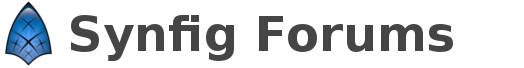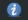# How to find the (exact) height of a layer?

So I’ve got a layer with a Shape in it. How do I find out exactly how high it is, i.e., what y dimension the outer bounding box of the shape has?

I plan to use it is for tiling this Shape vertically and seamlessly. I’d have the following setup:

1. Group A
1. “Duplicate” layer
From = 0.00
To = 7.00
Step = 1.00
2. Group B
Scalar = (linked to the duplicate layer, above)
1. Shape

For the “???” I need to know exactly how high the Shape layer is.

Just take the Y coordinate of the highest vertex in your shape. Or did I misunderstand something?

Well, yes, this kind of works. I was looking for some kind of Width and Height indication, the way Inkscape has it:But I suppose you can always check the vertices and find out that way. If it’s a single layer as opposed to a group of some complicated calculations.

Thanks!

Well, as far as I know Synfig doesn’t display dimensions of the shape anywhere.
The closest thing is the “Info”panel (Menu->Window->Info) that display coordinates of the mouse cursor, which can be a bit faster but less precise than going into the list of vertices.

If it is a shape which doesn’t have any curve you can use this method, but this won’t work if the object has curves. Then you would have to calculate the maxima of the curve and then use the highest point. (Same for the lowest point)

1 Like